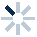# 3.7.2 必修1 项目七用随机投点法设计算法 学案21. Python  随机数产生模块－random
Python random 模块主要用于生成随机数。
random 模块实现了各种分布的伪随机数生成器。

from random import random            #导入random包中的random函数
a=random()                                         #直接引用random函数
b=random()
print(a,b)

import  random              # 导入 random 包
a=random.random()      #产生一个[0，1）随机数赋值给a
b=random.random()      #产生一个[0，1）随机数赋值给b
print(a,b)

[  拓广知识： ]
random随机数模块有许多，常用的有：
1.random.randint(a,b)

a：随机区间的开始值,整数

b：随机区间的结束值，随机数包含结束值整数

2.random.uniform(a,b)

a：随机区间的开始值，整数或浮点数

b：随机区间的结束值，随机数包含结束值，整数或浮点数

import  random
a=random.random()
b=random.randint(1,10)
c=random.uniform(1,10)
print("a=",a,"b=",b,"c=",c)

*
A. a= 0.65.. b= 9 c= 7.58..
B.a= 0.48.. b= 6 c= 5.43..
C.a= 0.78.. b= 8 c= 0.69..
D.a= 0.38.. b= 10 c= 6.59..
2. 下列程序中定义函数语句定义不正确的是（     ）
import  math
import  random
a=random.randint(100,1000)
b=randint(100,1000)
c=math.sqrt(a)
d=math.sqrt(b)
print(a,b,c,d)*
A. a=random.randint(100,1000)
B. b=randint(100,1000)
C. c=math.sqrt(a)
D. d=math.sqrt(b)
3. python变量赋值：
python中的变量在使用前必须赋值，

1>单行定义变量
counter = 100          # 整型变量
miles = 1000.0         # 浮点型变量
name = "python"      # 字符串

2>同时为多个变量赋值。

a = b = c = 1                   #三个变量同量赋相同的值
a, b, c = 1, 2, "python"  #两个整型数1，2分别赋给变量a,b,字符串对象“python”赋给变量c

*
A. a:b:c=1:2:3
B. a,b,c=1,2,python
C. a/b/c=1/2/3
D. a,b,c=1,2,"python"
4.1.输入总的投点数 darts
2.令hits=0;
3.循环i从0到dars-1,step1; 输出圆周率的近似值
3.1 获取x,y的随机值
3.2 如果x2+y2<1,则hits增加1;
4.计算圆周率的近似值;
5.输出圆周率的近似值;5. P101活动7 改写程序：计算并输出不同精确度值pi的值及输出运算时间。*